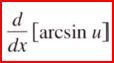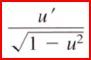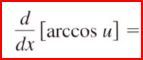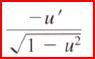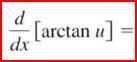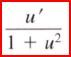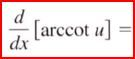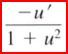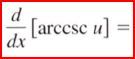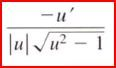• Shuffle
Toggle On
Toggle Off
• Alphabetize
Toggle On
Toggle Off
• Front First
Toggle On
Toggle Off
• Both Sides
Toggle On
Toggle Off
Toggle On
Toggle Off
Front

## Card Range To Study

throughPlay buttonPlay buttonProgress

1/19

Click to flip

Use LEFT and RIGHT arrow keys to navigate between flashcards;

Use UP and DOWN arrow keys to flip the card;

H to show hint;

 what is the domain & range of: Y = arcsin x iff sin y = x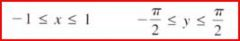(left to right: domain, range) What is the domain and range of: y = arctan x iff tan y = x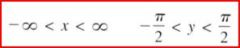(from left to right: domain, range) What is the domain and range of: y = arccot x iff cot y = x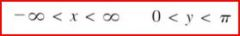(from left to right: domain, range) what is the domain and range of: y = arcsec x iff sec y = x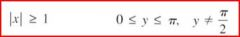(from left to right: domain, range) What is the domain and range of: y = arccsc x iff csc y = x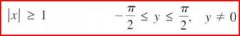(from left to right: domain, range) y = arcsin x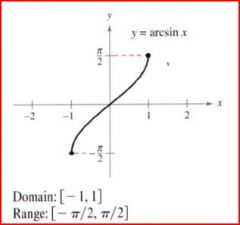y = arccos x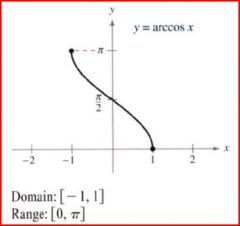y = arctan x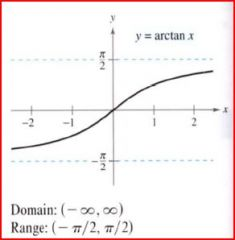y = arccsc x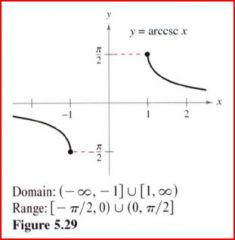y= arcsec x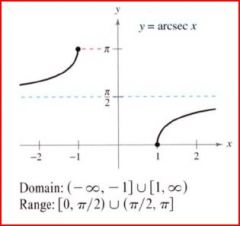y = arccot x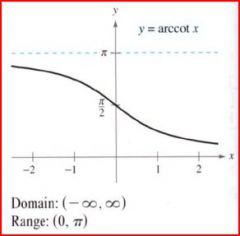sin(arcsin x) = x tan(arctan x) = x sec(arcsec x) = x